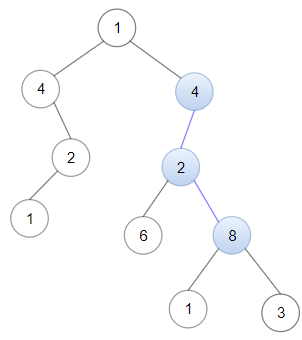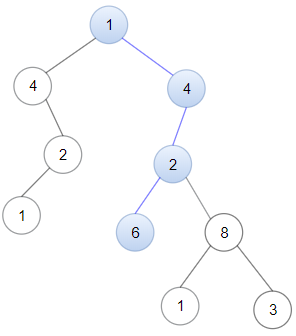# GeetCode Hub

Given a binary tree `root` and a linked list with `head` as the first node.

Return True if all the elements in the linked list starting from the `head` correspond to some downward path connected in the binary tree otherwise return False.

In this context downward path means a path that starts at some node and goes downwards.

Example 1:```Input: head = [4,2,8], root = [1,4,4,null,2,2,null,1,null,6,8,null,null,null,null,1,3]
Output: true
Explanation: Nodes in blue form a subpath in the binary Tree.
```

Example 2:```Input: head = [1,4,2,6], root = [1,4,4,null,2,2,null,1,null,6,8,null,null,null,null,1,3]
Output: true
```

Example 3:

```Input: head = [1,4,2,6,8], root = [1,4,4,null,2,2,null,1,null,6,8,null,null,null,null,1,3]
Output: false
Explanation: There is no path in the binary tree that contains all the elements of the linked list from `head`.
```

Constraints:

• The number of nodes in the tree will be in the range `[1, 2500]`.
• The number of nodes in the list will be in the range `[1, 100]`.
• `1 <= Node.val <= 100` for each node in the linked list and binary tree.

/** * Definition for singly-linked list. * public class ListNode { * int val; * ListNode next; * ListNode() {} * ListNode(int val) { this.val = val; } * ListNode(int val, ListNode next) { this.val = val; this.next = next; } * } */ /** * Definition for a binary tree node. * public class TreeNode { * int val; * TreeNode left; * TreeNode right; * TreeNode() {} * TreeNode(int val) { this.val = val; } * TreeNode(int val, TreeNode left, TreeNode right) { * this.val = val; * this.left = left; * this.right = right; * } * } */ class Solution { public boolean isSubPath(ListNode head, TreeNode root) { } }